## Count on in tens (Christmas)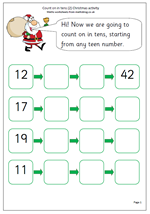We are rapidly approaching the Christmas season in schools, with plenty of work going on preparing concerts, nativities etc. but the maths work still needs to go on, so here are a couple of worksheets for Year 1 children with a Christmas feel to them.

They look at counting on in whole tens from any 2-digit number, with answers up to 100. It is important that children are confident and happy with counting in ones up to 100 before starting this and some children may still need a number square to help them with this. Counting on in tens also helps with the understanding of partitioning numbers into tens and units and it is important to ask children what is happening, both to the tens and the units.

Count on in tens (pg 2)

## New Maths Curriculum: Year 1 Geometry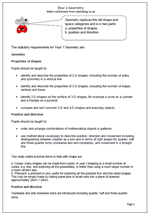Geometry replaces the old shape and space categories and is in two parts:

a. properties of shapes
b. position and direction.

The statutory requirements for Year 1 Geometry are:

Properties of shapes

Pupils should be taught to:

•    identify and describe the properties of 2-D shapes, including the number of sides and symmetry in a vertical line

•    identify and describe the properties of 3-D shapes, including the number of edges, vertices and faces

•    identify 2-D shapes on the surface of 3-D shapes, for example a circle on a cylinder and a triangle on a pyramid

•    compare and sort common 2-D and 3-D shapes and everyday objects.

Position and direction

Pupils should be taught to:

•    order and arrange combinations of mathematical objects in patterns

•    use mathematical vocabulary to describe position, direction and movement including distinguishing between rotation as a turn and in terms of right angles for quarter, half and three-quarter turns (clockwise and anti-clockwise), and movement in a straight line.

Two really useful practical items to help with shape are:

a. Cubes: many shapes can be made from cubes. In year 2 keeping to a small number of cubes, e.g. four, and exploring all the possibilities, is better than using a much larger number in a more random way.
b. Pinboard: a pinboard is very useful for exploring all the possible four and five sided shapes. This may be simply made by nailing panel pins or small nails into a piece of plywood approximately 24cm × 24cm.

Position and direction
Clockwise and anti-clockwise turns are introduced including quarter, half and three-quarter turns.

Year 1 Geometry: statutory requirements

## New Maths Curriculum: Year 1 Measurement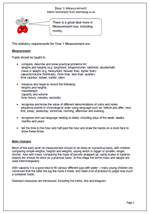Here are the statutory requirements for Measurement in Year 1. There is a great deal more in Measurement now, including money and time.

Measurement

Pupils should be taught to:

•    compare, describe and solve practical problems for:
lengths and heights (e.g. long/short, longer/shorter, tall/short, double/half)
mass or weight (e.g. heavy/light, heavier than, lighter than)
capacity/volume (full/empty, more than, less than, quarter)
time (quicker, slower, earlier, later)

•    measure and begin to record the following:
lengths and heights
mass/weight
capacity and volume
time (hours, minutes, seconds)

•    recognise and know the value of different denominations of coins and notes
sequence events in chronological order using language such as: before and after, next,
first, today, yesterday, tomorrow, morning, afternoon and evening

•    recognise and use language relating to dates, including days of the week, weeks, months and years

•    tell the time to the hour and half past the hour and draw the hands on a clock face to show these times.

Main changes

Much of the early work on measurement should to be done on a practical basis, with children comparing simple lengths, heights and weights, saying which is bigger or smaller, longer, shorter. Also with mass, comparing the mass of parcels wrapped up, using scales to balance objects etc should be done on a practical basis. At this stage the terms mass and weight are used interchangeably.

With capacity it is a good idea to fill various different jugs with water – many young children are convinced that the taller the jug the more it holds, and need a lot of practice to judge how much a container holds.

Standard measures are introduced, including the metre, litre and kilogram.

Year 1 Measurement statutory requirements

## New Maths Curriculum: Year 1 Fractions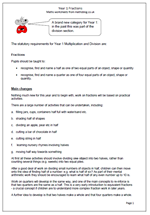Fractions is a brand new category for Year 1: in the past this was part of the division section.

The statutory requirements for Year 1 Multiplication and Division are:

Fractions

Pupils should be taught to:

•    recognise, find and name a half as one of two equal parts of an object, shape or quantity

•    recognise, find and name a quarter as one of four equal parts of an object, shape or quantity.

Main changes

Nothing much new for this year and to begin with, work on fractions will be based on practical activities.

There are a large number of activities that can be undertaken, including:

a.    filling jars, cups, containers half full with water/sand etc.

c.    dividing an apple, pear etc in half

d.    cutting a bar of chocolate in half

e.    cutting string in half

f.     learning nursery rhymes involving halves

g.    moving half way towards something

At first all these activities should involve dividing one object into two halves, rather than counting several things (e.g. sweets) into two equal piles.

After a good deal of work on dividing small numbers of objects in half, children can then move onto the idea of finding half of a number: e.g. what is half of six? As part of their mental arithmetic work they should be encouraged to learn what half of any even number up to 10 is.

Work on quarters will develop in the same way, and one of the main concepts to re-inforce is that two quarters are the same as a half. This is a very early introduction to equivalent fractions – a crucial concept if children are to understand more complex fraction work in later years.

A further idea to develop is that two halves make a whole and that four quarters make a whole.

Year 1 Fractions: statutory requirements

## Resource of the week: year 1 investigate addition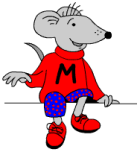Welcome to a new term and plenty of new maths material coming up over the next few months.

From our current resources here is a nice little investigation for young children which will show how well they can organise their thinking and work in a logical way.

The question is simple: how many different ways can you score 8 when throwing two dice?

It’s always a good idea to sit down with your children when doing this type of activity. In this way you can ask questions which will help them clarify their thoughts. The sheet is designed to be used as a record of results. At first they might just keep rolling the dice and adding up the totals until a total of 8 is achieved. Other children might dive straight in with some answers eg 4 + 4 makes 8.

A good question to ask at the end is “How do you know that you have got all the possibilities?

Free Y1 maths worksheet: Investigate dice

## Year 1 maths worksheet: numbers in order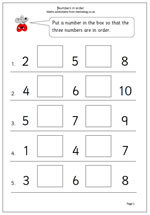I have just published a new worksheet on writing small numbers in order, for year 1 although suitable for younger children if ready. There is more than one possible answer on most of these which makes it a little trickier.
Many children learn to recite the numbers 1 to 10 without having any concept of what each number means so it is very important to show these numbers using practical ‘hands on’ equipment such as cubes, counters etc. and to understand one-to-one correspondence. To begin with some children will need to count up in ones from 1 to find each answer

There are also many activities which can help, such as tracing numbers in sand, tracing over numbers or using number cards to rearrange a small set of numbers in order.

Order numbers

## Counting across 100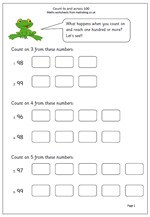The latest draft version of the Primary Maths Programme of Study includes counting to and across 100, which has usually been left until year 2. It is quite tricky and shouldn’t be covered until you are certain that children can count confidently up to 100.

Here is a worksheet aimed at helping with this, counting three, four or five more in steps of one and crossing the hundred boundary in each case. Thanks to urbrainy.com for letting me adapt one of their pages.

Count across one hundred

## Targets for Year 1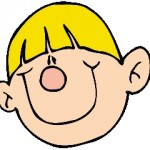To be able to successfully help your child with maths at home it is important to have a good idea of what is expected in school. This is a list of the targets expected of children by the end of Year 1 and most teachers will be planing their work around these. Of course this does not mean that all children will achieve these targets; some will find them impossible whilst many will be able to go on further. However, they do act as good starting point.

Counting and understanding number

By the end of year 1 children should

• know the number names (one, two, three etc) up to at least 20 and tens up to 100
(eg twenty, thirty, forty etc).

• be able to count up to 20 objects reliably. If the objects (eg buttons on a tray) are moved around they should be able to say that the total number has not changed without recounting. (Younger children will count them again if they have been moved around.)

• be able to read and write numerals up to 20
(eg 3, three, 4, four, 5 five, etc).

• be able to place numbers on a blank number line in the correct order.

• be able to say the number that is one more than or one less than any given number up to 20
(eg one less than 7).

• be able to say the number ten more than or ten less than a multiple of 10
(eg ten more than 30).

• recognise the equals sign (=) and understand it means “..is the same as..”

• use the terms, half and quarter in a practical sense
(eg give me a quarter of the orange).

Knowing and using number facts

By the end of year 1 children should

• know addition facts up to a total of 5
(eg 3 + 2).

• know all pairs of numbers with a total of 10
(eg 1 and 9, 2 and 8 etc).

• be able to work out subtraction facts from the addition facts they know.
(eg 5 -2 = 3 because 3 + 2 = 5)

• be beginning to count on and back in twos, fives and tens.

• know the doubles of all numbers up to 10
(eg double 6 is 12).

Calculating

By the end of year 1 children should

• know that addition can be done in any order.

• be able to write simple number sentences using the + and = signs
(eg 3 + 4 = 7).

• use practical methods (eg using counters) to help with addition of a 1-digit number to a 1-digit or 2-digit number
(eg 10 + 4 =).

• use informal written notes to help with addition

• understand that subtraction can mean ‘take away’.

• understand that subtraction can mean ‘find the difference between’.

• be able to use practical methods to help with subtraction of a 1-digit number from a 1-digit number
(eg using counters).

• use informal written notes to help with subtraction
(eg jotting down 5 circles and crossing out 3 for 5 – 3).

• be able to write simple number sentences using the – and = signs
(eg 7 – 4 = 3).

• understand and use the vocabulary related to addition and subtraction, including: more, add, sum, total, altogether, equals, =, take away, subtract, difference between, how much more/less, how many are left?

• be able to combine groups of 2, 5 and 10 in a practical situation
(eg combining marbles into groups of 5 marbles).

• be able to share a group of objects into 2 equal parts
(eg sweets shared equally).

Understanding shape

By the end of year 1 children should

• be able to visualise and name common 2-D shapes, including circle, triangle, rectangle and square. This includes activities such as “Find a shape which has 3 corners and 3 sides”.

• be able to visualise and name common 3-D shapes, including, cube, cuboid, sphere, cone and cylinder. This includes activities such as describing the properties of a shape by the feel of it, before seeing it.

• be able to make patterns and pictures with 2-D shapes and make models with 3-D shapes such as Lego.

• begin to describe the position of shapes, using terms such as: over, under, underneath, above, below, outside, inside, in front, behind, beside, before, after, next to, opposite, between, middle, centre, edge, corner, top, bottom, side. This is where activities such as moving furniture around a toy house are very important or placing counters on a board game.

• be using everyday language to describe direction, such as: left, right, up, down, forwards, backwards, sideways, across, along, through, to, from, towards, away from.

• begin to be able to describe movements in a straight line and turning. At first turning will only be by whole and half turns but it is important to recognise shapes that turn about a point, such as a pair of scissors.

• Once again it must be emphasised that most of this work is done orally with practical equipment. Toys, board games, may seem like playing to us but are invaluable learning experiences.

Measuring

By the end of year 1 children should
• understand and use the basic vocabulary related to length, mass and capacity.
For length: long, short, tall, high, low, wide, narrow, deep, shallow, thick, thin, far, near and words of comparison eg longer.
For mass: weight, weighs, heavy light, balances.
For capacity: full, empty, half full, holds.

• be able to make direct comparisons between two items eg comparing the length of two pencils, the weight of two parcels, the capacity of two cups. A set of balance scales is very useful for this kind of work.

• begin to use non-standard measures
(eg the jug holds 6 cups of water, the table is about 7 rulers long).

• be making sensible estimates and guesses related to length, mass and capacity. Standard units such as cm, km, kg are not used at this stage.

Using and applying maths

By the end of year 1 children should

• solve problems involving counting, addition, subtraction, doubling, halving, measures and money
(eg a toy shop, paying and giving change with numbers up to 10).

• describe and solve simple puzzles or problems
(eg How many ways can you score 4 rolling two dice?).

• sort information, shapes or objects and display the results using pictures
(eg sort cubes by colour).

• describe simple patterns involving numbers or shapes
(eg continue a pattern of repeated squares and circles).

• describe ways of solving a puzzle or problem orally
(eg talk about or draw what coins could be used to make 6p).

## Resource of the week: sorting shapes in Year 1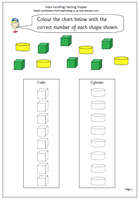One of the earliest stages of data handling is to be able to sort and a lot of practical work can be done, such as tidying up a drawer of pens, pencils, paper clips etc. Don’t underestimate this type of practical activity as it is important for children learning to group and order objects.

The next stage is to be able to record the results and here we have a simple page where the two sets of shapes can be sorted and recorded on the columns.

The outlines of the cylinders and cubes are provided and it is just a matter of counting the number of each shape and recording by colouring the correct number on the columns.

The task can be extended by providing real cubes, keeping to two or three colours and recording how many there are in columns.

This page can be found in our Handling Data section for Year 1.

Sorting shapes 1

## Year 1 maths worksheet: subtraction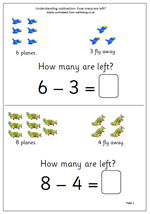It has been a while since I published anything for year 1, so here are a couple of pages on understanding subtraction. They look very straightforward but there are a number of concepts involved here:

Firstly, understanding that the phrase, ‘How many are left’ .

Secondly, relating subtraction to ‘taking away’.

Thirdly, the answer can be found by counting up from a number.

Fourthly, the answer can be found by counting back from the larger number.

Finally, that the minus sign means subtract.

Thanks to urbrainy.com for letting me use these two pages.

How many are left?